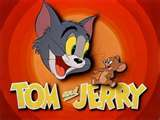# How Much Is 16 Percent Of 28?

4.48....
thanked the writer.
16  percent of 28 is 4.48
thanked the writer.
16 percent of 28..... 28
x.16
____
168
280
____
4.48
thanked the writer.
Generally 16 per cent of 28 is 4.48. You start off the working with, 16 multiplied by 28 upon hundred and solve it accordingly. Percentage basically is a method of expressing a figure as a fraction of 100.

It is normally signified by applying the percentage sign, like 65 per cent is equal to 65/100 or simply 0.65. Percentages are generally applied to indicate how big a particular quantity is in comparison to another. The initial quantity generally symbolizes a section of, or a modification in the latter quantity. While percentages are generally applied to state figures amid zero and one, every dimensionless proportionality can be stated as percentage.

Percentages are appropriately applied to state fractions of the total, like 50% means 50/100 or "half". Owing to inconsistent application, it is generally not clear from the circumstance what a percentage is relative to.thanked the writer.